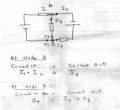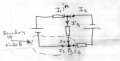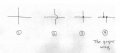# Kirchoffs Theory

#### John54321

Joined Apr 1, 2015
39
Hi
Im struggling with these questions would be very greatful of any advice please

#### WBahn

Joined Mar 31, 2012
26,398
Yep, those are questions alright.

The first step is to show YOUR best effort to solve YOUR problems. Then we can see what you are doing right and where you are having problems and offer hints and suggestions to lead you back onto the right path.

#### John54321

Joined Apr 1, 2015
39
Hi WBahn
You will have to bear with me I have 1 assignment on Ac Dc theory to do on a self study course. So this is all new to me does my labelling of diagram look ok so far ? im struggling the next move from here for the resultant current in R2.

#### studiot

Joined Nov 9, 2007
4,998
Let us start at the beginning of the question.

You have copied their diagram, it's true.

But before you can successfully label things as they ask you need to know Kirchoffs laws.

Can you state them?

In particular can you state the current law?

#### John54321

Joined Apr 1, 2015
39
Yeh sum of junction going in = sum out
I1 + I2 =I3
loop rule = 0

#### studiot

Joined Nov 9, 2007
4,998
OK I see two nodes (we call them nodes not junctions).

Step 1 label the nodes. A, B will do.

Step 2 For each branch at node A and node B insert a current and an assumed direction arrow.

Apply KCL to this node.

For Kirchoff's voltage law (which you didn't state)

Identify and label all the loops.
I see three loops.

Form KVL equations by applying KVL to each loop.

#### John54321

Joined Apr 1, 2015
39
KCL?

The current already shows the direction 17v and 34v

ive labelled 2 loops 3rd loop is the complete outside loop I take it

#### studiot

Joined Nov 9, 2007
4,998
KCL = Kirchoff's Current Law

The sum of the currents entering a node = the sum of the currents leaving.

This is true for every node in the circuit.

So you will obtain an equation connecting currents for each and every node.

How many equations will that yield in this case?

Note also that KCL has no voltages in it.

KVL = Kirchoff's Voltage Law

The sum of the EMF voltages around any closed loop = The sum of the IR voltage drops in that loop.
You can obtain an equation for each and every loop in the circuit
Note that KVL conains both voltages and currents

How many equations are therefore in your circuit?

These are the basic forms of Kirchoff's laws.

Others here will cut corners and combine these with other ideas to form what are known as the mesh equations which also contain both voltage and current.
These are short cuts that are best studied once the long slog method is fully understood.

Here is a start on your circuit.

I have labelled the nodes A and B and shown the currents for Node A

Can you do the same for NodeB?#### John54321

Joined Apr 1, 2015
39
KCL = Kirchoff's Current Law

The sum of the currents entering a node = the sum of the currents leaving.

This is true for every node in the circuit.

So you will obtain an equation connecting currents for each and every node.

How many equations will that yield in this case?

Note also that KCL has no voltages in it.

KVL = Kirchoff's Voltage Law

The sum of the EMF voltages around any closed loop = The sum of the IR voltage drops in that loop.
You can obtain an equation for each and every loop in the circuit
Note that KVL conains both voltages and currents

How many equations are therefore in your circuit?

These are the basic forms of Kirchoff's laws.

Others here will cut corners and combine these with other ideas to form what are known as the mesh equations which also contain both voltage and current.
These are short cuts that are best studied once the long slog method is fully understood.

Here is a start on your circuit.

I have labelled the nodes A and B and shown the currents for Node A

Can you do the same for NodeB?View attachment 83279

#### John54321

Joined Apr 1, 2015
39

Would node B for the left loop 1 be i3 + i1 , loop 2 right side i3 + i2?

#### studiot

Joined Nov 9, 2007
4,998
Would node B for the left loop 1 be i3 + i1 , loop 2 right side i3 + i2?
I am working through this in excruciating detail with you because I think you teaching material is too condensed and that you have either not seen or the basics.

Don't worry once you have done it once you should not need to do it again in such detail and will be able to see how to condense the statements.

The quote above suggests you do not properly understand what a node or loop is, which is part of the trouble.
I have added nodeB to my diagram and three more currents for the three more branches.

It is very very very important to realise that the arrows represent a guess for the directions of the currents.
If the guess is wrong the current will simply come out negative.

We can discuss this as we go along and develop the diagram.•John54321

#### John54321

Joined Apr 1, 2015
39
Sorry I assumed there was only 3 branch's the centre i3 right side i2 left side i1

#### studiot

Joined Nov 9, 2007
4,998
Indeed we will find that I4 = I3 and I1 = I5 and I2 = I6

for this simple circuit.

Can you see why?

But that is not always the case.

A branch connects two nodes, with no other nodes in the path.

This is very important.

Last edited:

#### studiot

Joined Nov 9, 2007
4,998
Nodes.

I see you used the word 'junction'.
This implies a connection to me at any rate.
A connection is really a single point in the circuit.
There may be many connections or junctions at a node.

A node is a region of a circuit that is all at the same potential (voltage).
It is not usually a single point but spread out.

I have outlined the boundary of the B node in the circuit with a dashed line.Can you do this for the A node?

Talking of junctions,

When two wires cross in a drawing we have to indicate if there is a connection or not., as is fig1.
The oldfashioned way is show them definitely not connecting as in fig2
A more modern way is to show them definitely connecting as in fig3
This causes difficulty in some more complicated circuits and the correct implementation of this convention is in fig 4 where any connection has a maximum of three branches, so we can always identify the different branch currents.#### John54321

Joined Apr 1, 2015
39
The way i see it as 17v goes into R1 as a + current then goes down to R2 am i correct in thinking that the current goes into a resistore as a + current and out a - current ?

Node a
so I1 + I2 = I3

17 + 34 = 51

Node b

I4 = I5 + I6

34+ 17 = 51

#### studiot

Joined Nov 9, 2007
4,998
The way i see it as 17v goes into R1 as a + current then goes down to R2 am i correct in thinking that the current goes into a resistore as a + current and out a - current ?
No.

That is why I am trying starting from square 1 and trying to work through with you in a detailed way, since you say you are self studying this.

I am glad you realise that current passes through something though, many don't.

We haven't come to the voltages yet.

How are you getting on with the simple questions I asked?

This is not elementary circuit theory and looking at the next section in your question sheet, it gets quite advanced.

You will not succeed at that level without a good grasp of the basics, which I am trying to make clear by building up a bit at a time.

Last edited:

#### John54321

Joined Apr 1, 2015
39
Thanks for taking the time to explain but I can't see where you get the values from.

I will take a look at this in depth later. I noticed your from Somerset, I live in Hatch Beauchamp taunton.

#### studiot

Joined Nov 9, 2007
4,998
I didn't post any values, just some equations connecting three (six) currents ?

It is not a good idea to be too specific about your private details in open forum.
In particular you should never post your own Email here (as some do).

This forum boasts a PM - private messaging service you are advised to use that.

I also suggest you remove your Stanley Baker details.
My company, Studio T is based in Taunton.

We can continue when you are ready.

#### John54321

Joined Apr 1, 2015
39
Sorry Stanley baker no idea what you mean.

Node A boundary is the top right